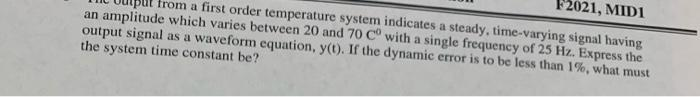# Question Solved1 Answerplease show work 2021, MIDI put from a first order temperature system indicates a steady, time-varying signal having an amplitude which varies between 20 and 70 Cº with a single frequency of 25 Hz. Express the output signal as a waveform equation, y(t). If the dynamic error is to be less than 1%, what must the system time constant be?DHDWKG The Asker · Mechanical EngineeringTranscribed Image Text: 2021, MIDI put from a first order temperature system indicates a steady, time-varying signal having an amplitude which varies between 20 and 70 Cº with a single frequency of 25 Hz. Express the output signal as a waveform equation, y(t). If the dynamic error is to be less than 1%, what must the system time constant be?
More
Transcribed Image Text: 2021, MIDI put from a first order temperature system indicates a steady, time-varying signal having an amplitude which varies between 20 and 70 Cº with a single frequency of 25 Hz. Express the output signal as a waveform equation, y(t). If the dynamic error is to be less than 1%, what must the system time constant be?